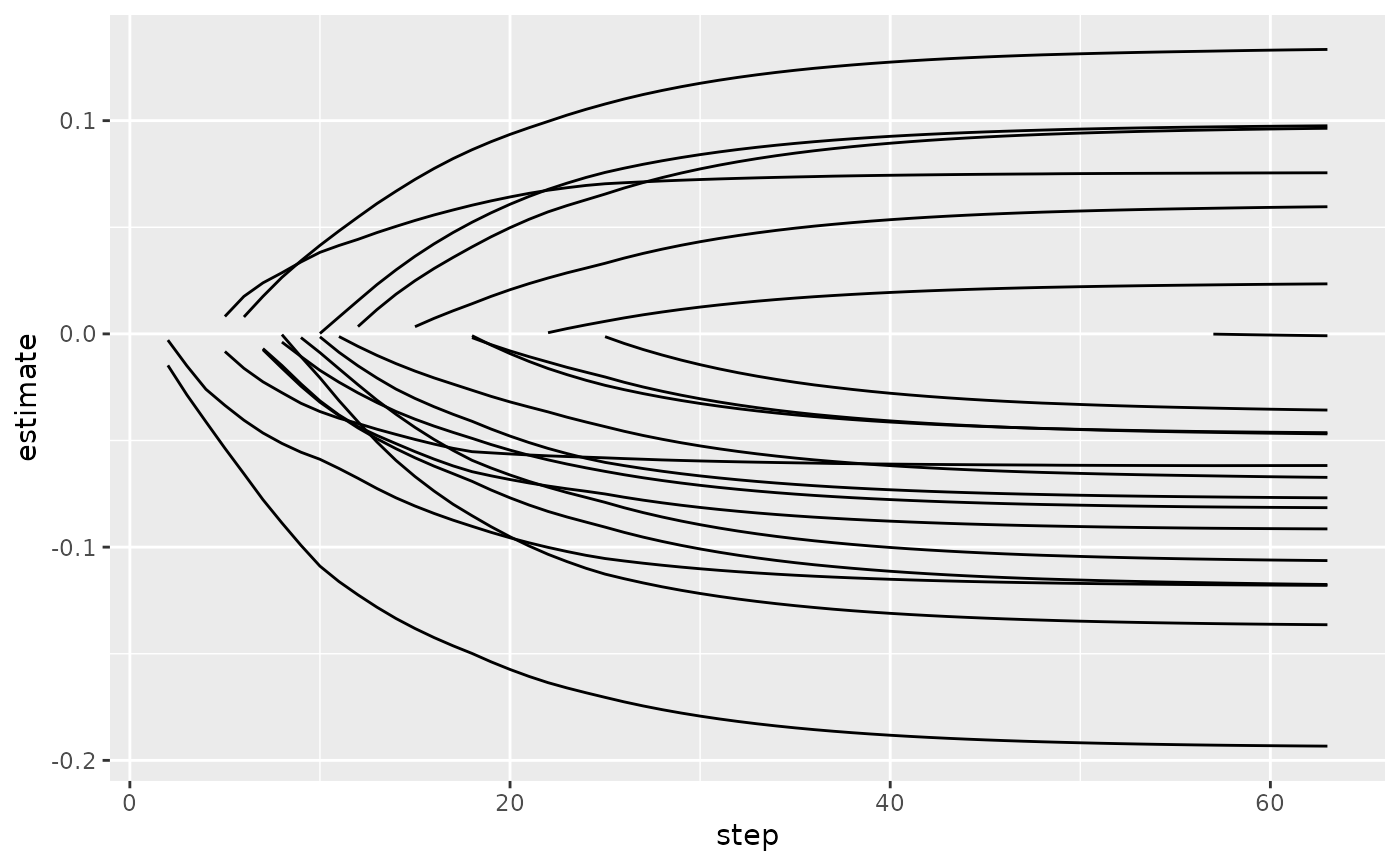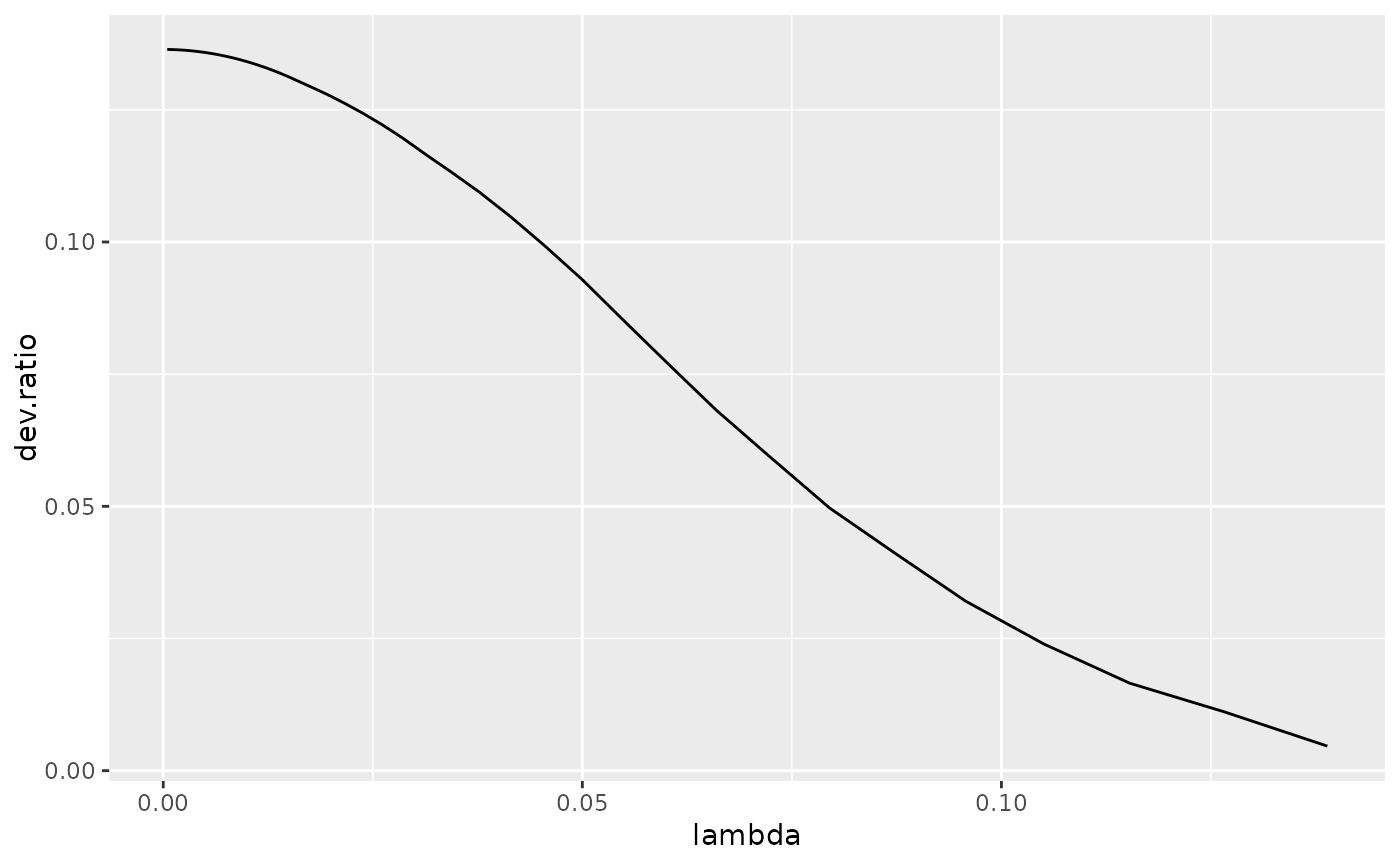Tidy summarizes information about the components of a model. A model component might be a single term in a regression, a single hypothesis, a cluster, or a class. Exactly what tidy considers to be a model component varies across models but is usually self-evident. If a model has several distinct types of components, you will need to specify which components to return.

## Usage

# S3 method for glmnet
tidy(x, return_zeros = FALSE, ...)

## Arguments

x

A glmnet object returned from glmnet::glmnet().

return_zeros

Logical indicating whether coefficients with value zero zero should be included in the results. Defaults to FALSE.

...

Additional arguments. Not used. Needed to match generic signature only. Cautionary note: Misspelled arguments will be absorbed in ..., where they will be ignored. If the misspelled argument has a default value, the default value will be used. For example, if you pass conf.lvel = 0.9, all computation will proceed using conf.level = 0.95. Two exceptions here are:

• tidy() methods will warn when supplied an exponentiate argument if it will be ignored.

• augment() methods will warn when supplied a newdata argument if it will be ignored.

## Details

Note that while this representation of GLMs is much easier to plot and combine than the default structure, it is also much more memory-intensive. Do not use for large, sparse matrices.

No augment method is yet provided even though the model produces predictions, because the input data is not tidy (it is a matrix that may be very wide) and therefore combining predictions with it is not logical. Furthermore, predictions make sense only with a specific choice of lambda.

tidy(), glmnet::glmnet()

Other glmnet tidiers: glance.cv.glmnet(), glance.glmnet(), tidy.cv.glmnet()

## Value

A tibble::tibble() with columns:

dev.ratio

Fraction of null deviance explained at each value of lambda.

estimate

The estimated value of the regression term.

lambda

Value of penalty parameter lambda.

step

Which step of lambda choices was used.

term

The name of the regression term.

## Examples


# load libraries for models and data
library(glmnet)

set.seed(2014)
x <- matrix(rnorm(100 * 20), 100, 20)
y <- rnorm(100)
fit1 <- glmnet(x, y)

# summarize model fit with tidiers + visualization
tidy(fit1)
#> # A tibble: 1,086 × 5
#>    term         step estimate lambda dev.ratio
#>    <chr>       <dbl>    <dbl>  <dbl>     <dbl>
#>  1 (Intercept)     1   -0.207 0.152    0
#>  2 (Intercept)     2   -0.208 0.139    0.00464
#>  3 (Intercept)     3   -0.209 0.127    0.0111
#>  4 (Intercept)     4   -0.210 0.115    0.0165
#>  5 (Intercept)     5   -0.210 0.105    0.0240
#>  6 (Intercept)     6   -0.210 0.0957   0.0321
#>  7 (Intercept)     7   -0.210 0.0872   0.0412
#>  8 (Intercept)     8   -0.210 0.0795   0.0497
#>  9 (Intercept)     9   -0.209 0.0724   0.0593
#> 10 (Intercept)    10   -0.208 0.0660   0.0682
#> # ℹ 1,076 more rows
glance(fit1)
#> # A tibble: 1 × 3
#>   nulldev npasses  nobs
#>     <dbl>   <int> <int>
#> 1    104.     255   100

library(dplyr)
library(ggplot2)

tidied <- tidy(fit1) %>% filter(term != "(Intercept)")

ggplot(tidied, aes(step, estimate, group = term)) +
geom_line()ggplot(tidied, aes(lambda, estimate, group = term)) +
geom_line() +
scale_x_log10()ggplot(tidied, aes(lambda, dev.ratio)) +
geom_line()# works for other types of regressions as well, such as logistic
g2 <- sample(1:2, 100, replace = TRUE)
fit2 <- glmnet(x, g2, family = "binomial")
tidy(fit2)
#> # A tibble: 947 × 5
#>    term         step estimate lambda dev.ratio
#>    <chr>       <dbl>    <dbl>  <dbl>     <dbl>
#>  1 (Intercept)     1    0.282 0.0906 -1.62e-15
#>  2 (Intercept)     2    0.281 0.0826  6.28e- 3
#>  3 (Intercept)     3    0.279 0.0753  1.55e- 2
#>  4 (Intercept)     4    0.277 0.0686  2.48e- 2
#>  5 (Intercept)     5    0.284 0.0625  4.17e- 2
#>  6 (Intercept)     6    0.293 0.0569  5.79e- 2
#>  7 (Intercept)     7    0.303 0.0519  7.39e- 2
#>  8 (Intercept)     8    0.314 0.0473  8.94e- 2
#>  9 (Intercept)     9    0.325 0.0431  1.03e- 1
#> 10 (Intercept)    10    0.336 0.0392  1.14e- 1
#> # ℹ 937 more rows